Function Repository Resource:

# FoliationClass

Generate the foliation set from a causal graph

Contributed by: Tom Lee, Jon Lederman
 ResourceFunction["FoliationClass"][data] returns the foliation set from the causal graph coordinate data.

## Details

The data input should be nodes, each represented as a triple with node index as first coordinate.
Nodes have a second coordinate representing time and are thus regarded as causal.
For example, the data set {{1, 0, 1}, {2, 0, 3}, {3, 2, 4}} represents three-point coordinate information of the triangular shaped causal graph. The first element of each set is the index of the point, eg. point 1, point 2, point 3. The second and third elements are the temporal and spacial coordinates, e.g. point 1 is the event at time=0, space=1.
Foliation is the process of categorizing points by time intervals where their time coordinates are included. For example, the data set {{1, 0, 1}, {2, 0, 3}, {3, 2, 4}} the point 1 and 2 are on the same temporal line (foliation class), and the point 3 is in later different foliation class.
The time intervals of the foliation are 1.
Causal graphs inputs can be generated using the resource function SimpleCausalGraphCoordinates.
The function returns the categorized point index where each element represents a node. For example, for the data set {{1, 0, 1}, {2, 0, 3}, {3,2,4}} the function returns {{1,2},{3}}.
By using different causal data set instead of using SimpleCausalGraphCoordinates function, one can generalize the causal graph structure with arbitrary rewriting rules.

## Examples

### Basic Examples

Find the foliation of three causal points in Euclidean coordinates:

 In:=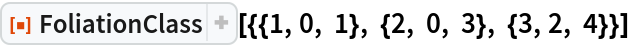Out=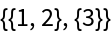Construct the simple triangular shape causal graph with 10 initial nodes:

 In:=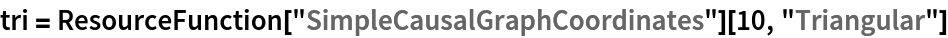Out=See the triangular shape:

 In:=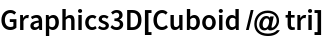Out=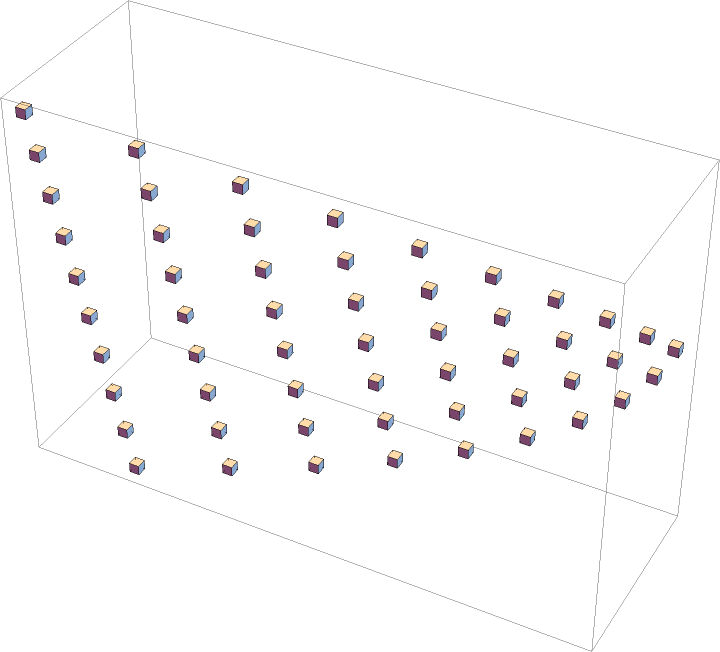Find the foliation:

 In:=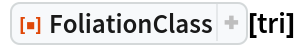Out=In:=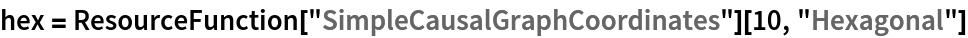Out=In:=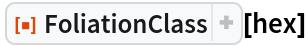Out=Tom Lee

## Version History

• 2.0.0 – 15 June 2022
• 1.0.0 – 07 June 2022